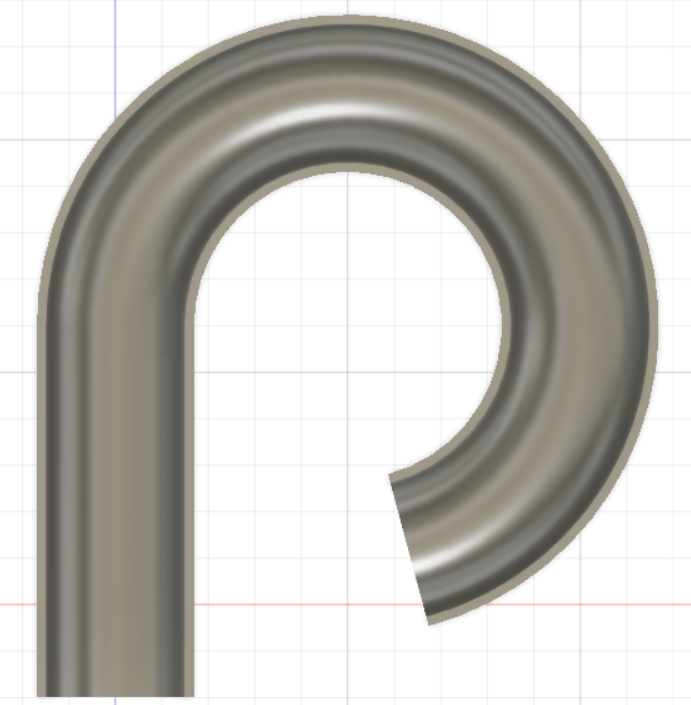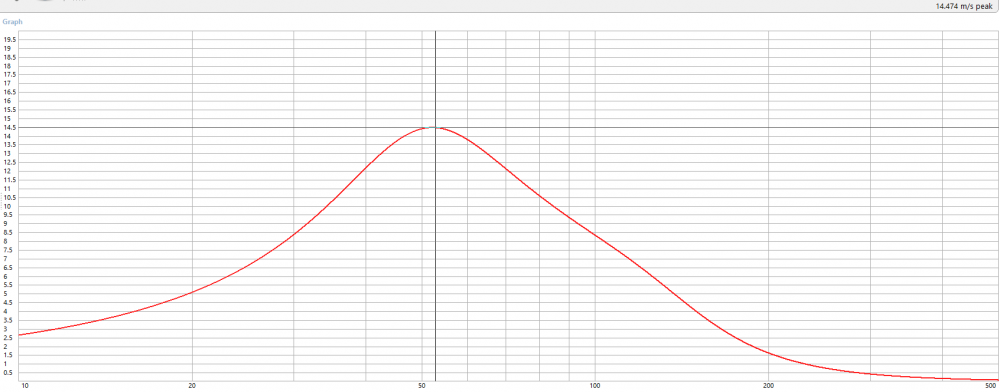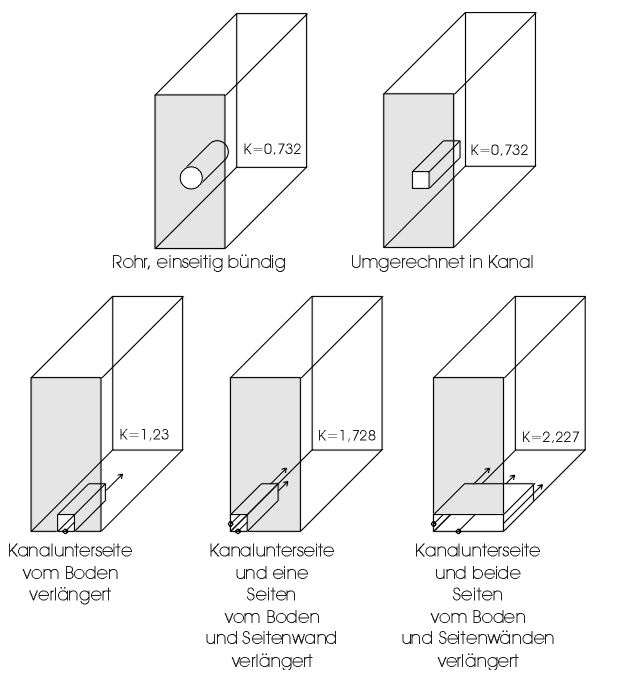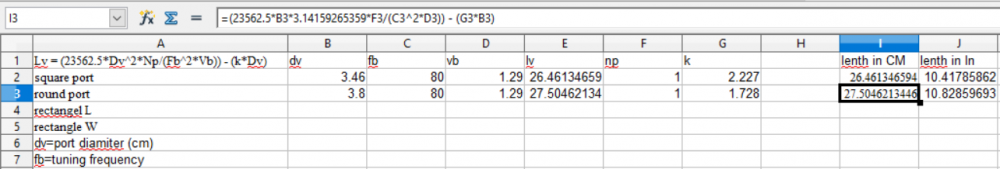#### Howdy, Stranger!

It looks like you're new here. If you want to get involved, click one of these buttons!

#### Who's Online (7)

Get familiar with the reaction system: Introducing the Reaction System

# Port Question

in DIY
I am modeling a small ported box and the port is getting long. To tune the box it needs to be 15.125 long by 1.5 ID.  Is there any better way to make the port than this? I will be printing this pending any warnings. The exit will get a flare with the router when it is complete.• Here is the port velocity at X-max according to Winisd.• I would minimize the bend radius as much as possible to prevent the outer portion of the column of air from suffering from frictional losses at high SPL.  If you don't play to utilize the woofer at high excursion, then it won't matter much.  But if you can minimize it, do it, as it won't cost anything.

On a different not, you can get more air mass to couple with the port mass by using two nearby enclosure walls, and perhaps an adapter to smoothly "connect" to the walls.  This will allow you to drastically shorten the port length without a penalty in shifting the Fb.  The bad part is that you have to figure this out empirically.  I did so, but I did it with a large slot port using 3-enclosure walls as the port walls themselves.   The port length needed  (for my 33Hz tuning) was 40% shorter than what modeling software calls for.  This was in my old JBL W10GTi box I had in my car back in the day.  I still have it in fact.  So I was able to use a very large port area, and still be able to tune low.  I'm not saying that percentage will be applicable with a circular port, but I would say you could attain a 20-30% reduction in required port length if you can fabricate a flare that couples smoothly and directly to the enclosure walls, so long as you're not close to the rear wall of the enclosure.
• edited April 18
+1 For small boxes, I do like using the walls and making a slot port.  I regularly miter PVC pipe for 1-90 elbow. I don't see anything wrong with your plan as long as you can trim it for tuning.
John H, thanks to JP I did get that email
• The port as drawn is the correct lenth and that radius is as large as I can fit in this box. The port will be 3d printed. I can easily cut it smaller if needed.
I'm not sure I understand the idea of using 2 walls to get the air mass to couple?
• The port end correction value (aka "k factor") changes for different types of slot ports.  The diagrams below (anyone want to translate the German?) give the different k factors for different port configurations.  Here's an excerpt from Troels Gravesen's website that gives the length equation:

Port Length:

The port length required to tune a volume of air to a specific frequency can be calculated by using the following equation:

Lv = (23562.5*Dv^2*Np/(Fb^2*Vb)) - (k*Dv), where:

Dv = port diameter (cm)

Fb = tuning frequency (Hz)

Vb = net volume (litres)

Lv = length of each port (cm)

Np = number of ports

k = end correction (normally 0.732)

The value for k, the end correction, can be fine-tuned by using the following values to derive the appropriate end correction figure for each end of the port, then adding them together:

Both ends flanged: k = 0.425 + 0.425 = 0.850

One end flanged, one end free: k = 0.425 + 0.307 = 0.732

Both ends free: k = 0.307 + 0.307 = 0.614

Normally, k=0.732 is assumed.• How do you shorten slot ports empirically (which I assume you fo by taking in box impedance measurements of the driver).
Typically the slot ports end up being part of the cabinet structure and would be glued in before measurements commences.
• I put the formula into an open office spreadsheet and having both ends flanged it shortens the length to 10.8. This makes the port much easier to manage. I can fit the port into the box with one 90* bend.